Name:    Practice Test 1B

Multiple Choice
Identify the choice that best completes the statement or answers the question.

1.

Which of the following statements concerning equal volumes of gases N2O and C3H8 , at the same temperature and pressure, is true?
 A) They have the same density B) They have the same number of moleucles C) The have the same number of atoms D) Both A and B

2.

What is the partial pressure of oxygen in a mixture containg 16 g of O2 and 16 g of SO2 gas and a total pressure of 660 mmHg?
 A) 440 mmHg B) 220 mmHg C) 330 mmHg D) 110 mmHg

3.

Based on the reaction shown below, which of the following statements is correct?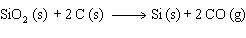A) Silicon is reduced and oxygen is oxidized B) Oxygen is reduced and carbon is oxidized C) Silicon is oxidized, and carbon is reduced D) Silicon is reduced and carbon is oxidized

4.

A 5.0-L sample of Ar gas at constant temperature and a pressure of 0.25 atm is compressed to a volume of 5.0 mL.  What is the final pressure?
 A) 250 atm B) 0.025 atm C) 25 atm D) 2.5 atm

5.

What volume of 1.00 M sodium hydroxide is required to react completely with 50.0 mL of 3.00 M sulfuric acid?
 A) 50.0 mL B) 300. mL C) 200. mL D) 150. mL

6.

A sample of Xe takes 75 seconds to effuse out of a container.  An unknown gas takes 37 seconds to effuse out of the identical container under identical conditions.  What is the most likelly identity of the unknown gas?
 A) O2 B) Br2 C) He D) Kr

7.

A compound contains 40.0% C, 6.7% H, and 53.3% O, with a molar mass of 60.0 g.  What is the molecular formula for this compound?
 A) C3H8O B) CH2O C) C2H4O D) C2H4O2

8.

A compound of chromium and silicon contains 73.52% by mass chromium.  What is its empirical formula?
 A) Cr3Si2 B) CrSi3 C) Cr2Si3 D) Cr3Si

9.

A 5.54-g sample of vinegar was neutralized by 30.10 mL of 0.100 M NaOH.  What is the mass percent of acetic acid in vinegar?
 A) 9.23 % B) 0.184 % C) 3.26 % D) 5.43 %

10.

What is the final pressure of the system when the valve between the two bulbs is opened in the diagram below?  (Assume constant temperature)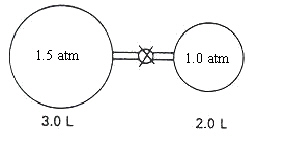A) 1.3 atm B) 2.0 atm C) 2.5 atm D) 0.50 atm

11.

Which of the following can act both as an oxidizing and a reducing agent?
 A) NH3 B) NO C) HClO4 D) H2S

12.

Which of the following are redox reactions?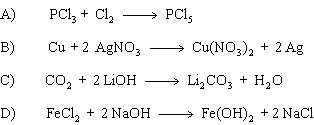A) A only B) A and B C) A, B and C D) A, B, C and D

13.

Which of the following statements is correct concerning the reaction shown below: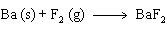A) The flourine atom is gaining electrons, therefore it is oxidized. B) The barium atom is gaining electrons, therefore it is oxidized. C) The fluorine atom is losing electrons, therefore it is reduced. D) The barium atom is losing electrons, therefore it is oxidized.

14.

A compound of chromium and silicon contains 73.52% by mass chromium.  What is its empirical formula?
 A) Cr3Si B) Cr3Si2 C) CrSi3 D) Cr2Si3

15.

If the formula for barium cerate is BaCeO3, what is the correct formula for ferric cerate?
 A) Fe3CeO3 B) Fe3(CeO3)2 C) Fe2(CeO3)3 D) FeCeO3

16.

A 3.00-mol sample of each reactant will produce what volume of N2O at STP, as shown below:A) 22.4 L B) 67.2 L C) 33.6 L D) 44.8 L

17.

Which of the following is the correct sequence of coefficients when the equation below is balanced:

___H3PO4  +  ___ MgCO3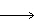___ Mg3(PO4)2 + ___ CO2 + ___ H2O
 A) 3, 1, 3, 2, 3 B) 2, 2, 1, 2, 3 C) 2, 3, 1, 3, 2 D) 2, 3, 1, 3, 3

18.

The oxidation number of sulfur in CaS2O3 is
 A) –4 B) +4 C) –2 D) +2

19.

The partial pressures of CH4 , N2 and O2 were found to be 100, 450, and 200 mmHg respectively.  What is the mole fraction of N2 in the mixture?
 A) 0.267 B) 0.333 C) 0.75 D) 0.6

20.

What is the molarity of a solution prepared by dissolving 7.31 g of Na2SO4 in enough water to make 225 mL of solution?
 A) 3.11 M B) 1.64 M C) 0.136 M D) 0.229 M